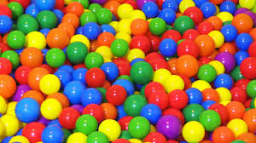# 20 balls

20 colored balls in a bag:
Four red
Seven green
Nine yellow
What is the probability of picking a yellow ball?

p =  0.45

### Step-by-step explanation:Did you find an error or inaccuracy? Feel free to write us. Thank you!

Tips for related online calculators
Need help calculating sum, simplifying, or multiplying fractions? Try our fraction calculator.
Would you like to compute the count of combinations?